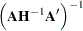Covariance Matrix of Parameter Estimates

For unconstrained estimates (no active bounds), the covariance matrix of the parameter estimates is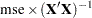for the gradient, Marquardt, and Gauss methods and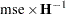for the Newton method. Recall thatis the matrix of the first partial derivatives of the nonlinear model with respect to the parameters. The matrices are evaluated at the final parameter estimates. The mean squared error, the estimate of the residual variance, is computed as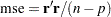whereis the number of nonmissing (used) observations andis the number of estimable parameters. The standard error reported for the parameter estimates is the square root of the corresponding diagonal element of this matrix. If you specify a value for the residual variance with the SIGSQ= option, then that value replacesin the preceding expressions.

Now suppose that restrictions or bounds are active. Equality restrictions can be written as a vector function,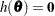. Inequality restrictions are either active or inactive. When an inequality restriction is active, it is treated as an equality restriction.

Assume that the vector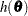contains the current active restrictions. The constraint matrix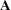is then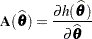The covariance matrix for the restricted parameter estimates is computed as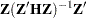where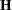is the Hessian (or approximation to the Hessian) and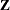collects the last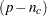columns of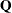from an LQ factorization of the constraint matrix. Further,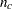is the number of active constraints, anddenotes the number of parameters. See Gill, Murray, and Wright (1981) for more details about the LQ factorization. The covariance matrix for the Lagrange multipliers is computed as